# Mahalanobis Distance – Understanding the math with examples (python)Mahalanobis distance is an effective multivariate distance metric that measures the distance between a point and a distribution. It is an extremely useful metric having, excellent applications in multivariate anomaly detection, classification on highly imbalanced datasets and one-class classification. This post explains the intuition and the math with practical examples on three machine learning use cases.Mahalanobis Distance – Understanding the Math and Applications. Photo by Greg Nunes.

## Content

1. Introduction
2. What’s wrong with using Euclidean Distance for Multivariate data?
3. What is Mahalanobis Distance?
4. The math and intuition behind Mahalanobis Distance
5. How to compute Mahalanobis Distance in Python
6. Usecase 1: Multivariate outlier detection using Mahalanobis distance
7. Usecase 2: Mahalanobis Distance for Classification Problems
8. Usecase 3: One-Class Classification
9. Conclusion

## 1. Introduction

Mahalanobis distance is an effective multivariate distance metric that measures the distance between a point (vector) and a distribution.

It has excellent applications in multivariate anomaly detection, classification on highly imbalanced datasets and one-class classification and more untapped use cases.

Considering its extremely useful applications, this metric is seldom discussed or used in stats or ML workflows. This post explains the why and the when to use Mahalanobis distance and then explains the intuition and the math with useful applications.

## 2. What’s wrong with using Euclidean Distance for Multivariate data?

Let’s start with the basics. Euclidean distance is the commonly used straight line distance between two points.

If the two points are in a two-dimensional plane (meaning, you have two numeric columns (p) and (q)) in your dataset), then the Euclidean distance between the two points (p1, q1) and (p2, q2) is: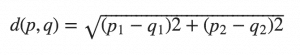This formula may be extended to as many dimensions you want: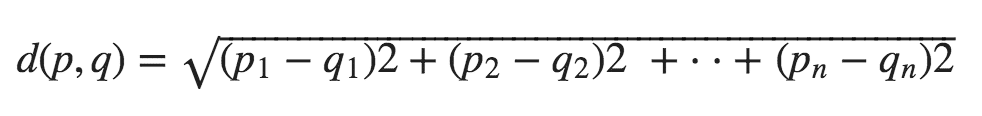Well, Euclidean distance will work fine as long as the dimensions are equally weighted and are independent of each other.

What do I mean by that?

Let’s consider the following tables: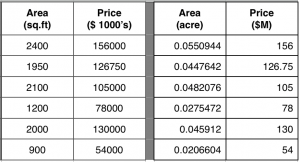The two tables above show the ‘area’ and ‘price’ of the same objects. Only the units of the variables change.

Since both tables represent the same entities, the distance between any two rows, point A and point B should be the same. But Euclidean distance gives a different value even though the distances are technically the same in physical space.

This can technically be overcome by scaling the variables, by computing the z-score (ex: (x – mean) / std) or make it vary within a particular range like between 0 and 1.

But there is another major drawback.

That is, if the dimensions (columns in your dataset) are correlated to one another, which is typically the case in real-world datasets, the Euclidean distance between a point and the center of the points (distribution) can give little or misleading information about how close a point really is to the cluster.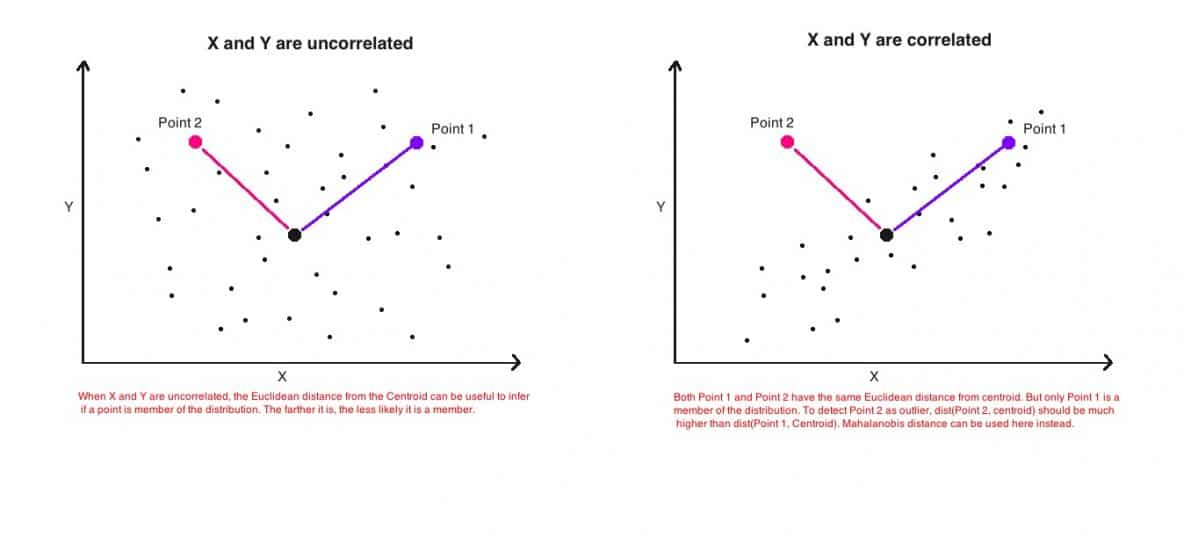The above image (on the right) is a simple scatterplot of two variables that are positively correlated with each other. That is, as the value of one variable (x-axis) increases, so does the value of the other variable (y-axis).

The two points above are equally distant (Euclidean) from the center. But only one of them (blue) is actually more close to the cluster, even though, technically the Euclidean distance between the two points are equal.

This is because, Euclidean distance is a distance between two points only. It does not consider how the rest of the points in the dataset vary. So, it cannot be used to really judge how close a point actually is to a distribution of points.

What we need here is a more robust distance metric that is an accurate representation of how distant a point is from a distribution.

## 3. What is Mahalanobis Distance?

Mahalonobis distance is the distance between a point and a distribution. And not between two distinct points. It is effectively a multivariate equivalent of the Euclidean distance.

It was introduced by Prof. P. C. Mahalanobis in 1936 and has been used in various statistical applications ever since. However, it’s not so well known or used in the machine learning practice. Well, let’s get into it.

So computationally, how is Mahalanobis distance different from Euclidean distance?

1. It transforms the columns into uncorrelated variables
2. Scale the columns to make their variance equal to 1
3. Finally, it calculates the Euclidean distance.

The above three steps are meant to address the problems with Euclidean distance we just talked about. But how?

Let’s look at the formula and try to understand its components.

## 4. The math and intuition behind Mahalanobis Distance

The formula to compute Mahalanobis distance is as follows: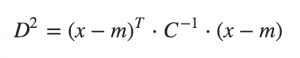where,
- D^2        is the square of the Mahalanobis distance.
- x          is the vector of the observation (row in a dataset),
- m          is the vector of mean values of independent variables (mean of each column),
- C^(-1)     is the inverse covariance matrix of independent variables. 

So, how to understand the above formula?

Let’s take the (x – m)^T . C^(-1) term.

(x – m) is essentially the distance of the vector from the mean. We then divide this by the covariance matrix (or multiply by the inverse of the covariance matrix).

If you think about it, this is essentially a multivariate equivalent of the regular standardization (z = (x – mu)/sigma). That is, z = (x vector) – (mean vector) / (covariance matrix).

So, What is the effect of dividing by the covariance?

If the variables in your dataset are strongly correlated, then, the covariance will be high. Dividing by a large covariance will effectively reduce the distance.

Likewise, if the X’s are not correlated, then the covariance is not high and the distance is not reduced much.

So effectively, it addresses both the problems of scale as well as the correlation of the variables that we talked about in the introduction.

## 5. How to compute Mahalanobis Distance in Python

import pandas as pd
import scipy as sp
import numpy as np

filepath = 'https://raw.githubusercontent.com/selva86/datasets/master/diamonds.csv'
df = pd.read_csv(filepath).iloc[:, [0,4,6]]
df.head()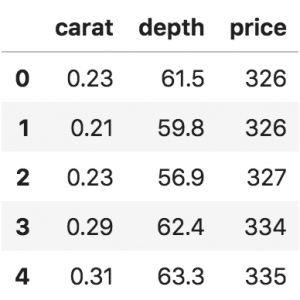Let’s write the function to calculate Mahalanobis Distance.

def mahalanobis(x=None, data=None, cov=None):
"""Compute the Mahalanobis Distance between each row of x and the data
x    : vector or matrix of data with, say, p columns.
data : ndarray of the distribution from which Mahalanobis distance of each observation of x is to be computed.
cov  : covariance matrix (p x p) of the distribution. If None, will be computed from data.
"""
x_minus_mu = x - np.mean(data)
if not cov:
cov = np.cov(data.values.T)
inv_covmat = sp.linalg.inv(cov)
left_term = np.dot(x_minus_mu, inv_covmat)
mahal = np.dot(left_term, x_minus_mu.T)
return mahal.diagonal()

df_x = df[['carat', 'depth', 'price']].head(500)
df_x['mahala'] = mahalanobis(x=df_x, data=df[['carat', 'depth', 'price']])
df_x.head()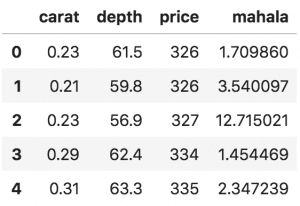## 6. Usecase 1: Multivariate outlier detection using Mahalanobis distance

Assuming that the test statistic follows chi-square distributed with ‘n’ degree of freedom, the critical value at a 0.01 significance level and 2 degrees of freedom is computed as:

# Critical values for two degrees of freedom
from scipy.stats import chi2
chi2.ppf((1-0.01), df=2)
#> 9.21


That mean an observation can be considered as extreme if its Mahalanobis distance exceeds 9.21.

If you prefer P values instead to determine if an observation is extreme or not, the P values can be computed as follows:

# Compute the P-Values
df_x['p_value'] = 1 - chi2.cdf(df_x['mahala'], 2)

# Extreme values with a significance level of 0.01
df_x.loc[df_x.p_value < 0.01].head(10)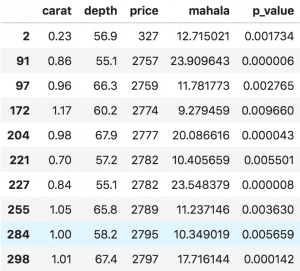If you compare the above observations against rest of the dataset, they are clearly extreme.

## 7. Usecase 2: Mahalanobis Distance for Classification Problems

Mahalanobis distance can be used for classification problems. A naive implementation of a Mahalanobis classifier is coded below. The intuition is that, an observation is assigned the class that it is closest to based on the Mahalanobis distance.

Let’s see an example implementation on the BreastCancer dataset, where the objective is to determine if a tumour is benign or malignant.

df = pd.read_csv('https://raw.githubusercontent.com/selva86/datasets/master/BreastCancer.csv',
usecols=['Cl.thickness', 'Cell.size', 'Marg.adhesion',
'Epith.c.size', 'Bare.nuclei', 'Bl.cromatin', 'Normal.nucleoli',
'Mitoses', 'Class'])

df.dropna(inplace=True)  # drop missing values.
df.head()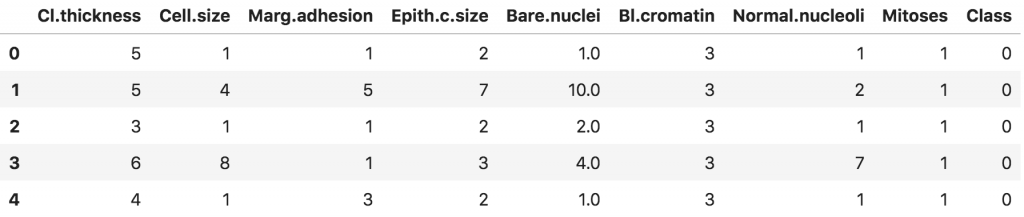Breast Cancer Dataset

Let’s split the dataset in 70:30 ratio as Train and Test. And the training dataset is split into homogeneous groups of ‘pos'(1) and ‘neg'(0) classes. To predict the class of the test dataset, we measure the Mahalanobis distances between a given observation (row) and both the positive (xtrain_pos) and negative datasets(xtrain_neg).

Then that observation is assigned the class based on the group it is closest to.

from sklearn.model_selection import train_test_split
xtrain, xtest, ytrain, ytest = train_test_split(df.drop('Class', axis=1), df['Class'], test_size=.3, random_state=100)

# Split the training data as pos and neg
xtrain_pos = xtrain.loc[ytrain == 1, :]
xtrain_neg = xtrain.loc[ytrain == 0, :]


Let’s build the MahalanobiBinaryClassifier. To do that, you need to define the predict_proba() and the predict() methods. This classifier does not require a separate fit() (training) method.

class MahalanobisBinaryClassifier():
def __init__(self, xtrain, ytrain):
self.xtrain_pos = xtrain.loc[ytrain == 1, :]
self.xtrain_neg = xtrain.loc[ytrain == 0, :]

def predict_proba(self, xtest):
pos_neg_dists = [(p,n) for p, n in zip(mahalanobis(xtest, self.xtrain_pos), mahalanobis(xtest, self.xtrain_neg))]
return np.array([(1-n/(p+n), 1-p/(p+n)) for p,n in pos_neg_dists])

def predict(self, xtest):
return np.array([np.argmax(row) for row in self.predict_proba(xtest)])

clf = MahalanobisBinaryClassifier(xtrain, ytrain)
pred_probs = clf.predict_proba(xtest)
pred_class = clf.predict(xtest)

# Pred and Truth
pred_actuals = pd.DataFrame([(pred, act) for pred, act in zip(pred_class, ytest)], columns=['pred', 'true'])
print(pred_actuals[:5])


Output:

   pred  true
0     0     0
1     1     1
2     0     0
3     0     0
4     0     0


Let’s see how the classifier performed on the test dataset.

from sklearn.metrics import classification_report, accuracy_score, roc_auc_score, confusion_matrix
truth = pred_actuals.loc[:, 'true']
pred = pred_actuals.loc[:, 'pred']
scores = np.array(pred_probs)[:, 1]
print('AUROC: ', roc_auc_score(truth, scores))
print('\nConfusion Matrix: \n', confusion_matrix(truth, pred))
print('\nAccuracy Score: ', accuracy_score(truth, pred))
print('\nClassification Report: \n', classification_report(truth, pred))


Output:

AUROC:  0.9909743589743589

Confusion Matrix:
[[113  17]
[  0  75]]

Accuracy Score:  0.9170731707317074

Classification Report:
precision    recall  f1-score   support

0       1.00      0.87      0.93       130
1       0.82      1.00      0.90        75

avg / total       0.93      0.92      0.92       205


Mahalanobis distance alone is able to contribute to this much accuracy (92%).

## 8. Usecase 3: One-Class Classification

One Class classification is a type of algorithm where the training dataset contains observations belonging to only one class.

With only that information known, the objective is to figure out if a given observation in a new (or test) dataset belongs to that class.

You might wonder when would such a situation occur. Well, it’s a quite common problem in Data Science.

For example consider the following situation: You have a large dataset containing millions of records that are NOT yet categorized as 1’s and 0’s. But you also have with you a small sample dataset containing only positive (1’s) records. By learning the information in this sample dataset, you want to classify all the records in the large dataset as 1’s and 0’s.

Based on the information from the sample dataset, it is possible to tell if any given sample is a 1 or 0 by viewing only the 1’s (and having no knowledge of the 0’s at all).

This can be done using Mahalanobis Distance.

Let’s try this on the BreastCancer dataset, only this time we will consider only the malignant observations (class column=1) in the training data.

df = pd.read_csv('https://raw.githubusercontent.com/selva86/datasets/master/BreastCancer.csv',
usecols=['Cl.thickness', 'Cell.size', 'Marg.adhesion',
'Epith.c.size', 'Bare.nuclei', 'Bl.cromatin', 'Normal.nucleoli',
'Mitoses', 'Class'])

df.dropna(inplace=True)  # drop missing values.


Splitting 50% of the dataset into training and test. Only the 1’s are retained in the training data.

from sklearn.model_selection import train_test_split
xtrain, xtest, ytrain, ytest = train_test_split(df.drop('Class', axis=1), df['Class'], test_size=.5, random_state=100)

# Split the training data as pos and neg
xtrain_pos = xtrain.loc[ytrain == 1, :]


Let’s build the MahalanobisOneClassClassifier and get the mahalanobis distance of each datapoint in x from the training set (xtrain_pos).

class MahalanobisOneclassClassifier():
def __init__(self, xtrain, significance_level=0.01):
self.xtrain = xtrain
self.critical_value = chi2.ppf((1-significance_level), df=xtrain.shape-1)
print('Critical value is: ', self.critical_value)

def predict_proba(self, xtest):
mahalanobis_dist = mahalanobis(xtest, self.xtrain)
self.pvalues = 1 - chi2.cdf(mahalanobis_dist, 2)
return mahalanobis_dist

def predict(self, xtest):
return np.array([int(i) for i in self.predict_proba(xtest) > self.critical_value])

clf = MahalanobisOneclassClassifier(xtrain_pos, significance_level=0.05)
mahalanobis_dist = clf.predict_proba(xtest)

# Pred and Truth
mdist_actuals = pd.DataFrame([(m, act) for m, act in zip(mahalanobis_dist, ytest)], columns=['mahal', 'true_class'])
print(mdist_actuals[:5])

Critical value is:  14.067140449340169
mahal  true_class
0  13.104716           0
1  14.408570           1
2  14.932236           0
3  14.588622           0
4  15.471064           0


We have the Mahalanobis distance and the actual class of each observation.

I would expect those observations with low Mahalanobis distance to be 1’s.

So, I sort the mdist_actuals by Mahalanobis distance and quantile cut the rows into 10 equal sized groups. The observations in the top quantiles should have more 1’s compared to the ones in the bottom. Let’s see.

# quantile cut in 10 pieces
mdist_actuals['quantile'] = pd.qcut(mdist_actuals['mahal'],
q=[0, .10, .20, .3, .4, .5, .6, .7, .8, .9, 1],
labels=[1, 2, 3, 4, 5, 6, 7, 8, 9, 10])

# sort by mahalanobis distance
mdist_actuals.sort_values('mahal', inplace=True)
perc_truths = mdist_actuals.groupby('quantile').agg({'mahal': np.mean, 'true_class': np.sum}).rename(columns={'mahal':'avg_mahaldist', 'true_class':'sum_of_trueclass'})
print(perc_truths)

          avg_mahaldist  sum_of_trueclass
quantile
1              3.765496                33
2              6.511026                32
3              9.272944                30
4             12.209504                20
5             14.455050                 4
6             15.684493                 4
7             17.368633                 3
8             18.840714                 1
9             21.533159                 2
10            23.524055                 1


If you notice above, nearly 90% of the 1’s (malignant cases) fall within the first 40%ile of the Mahalanobis distance. Incidentally, all of these are lower than the critical value pf 14.05. So, let’s the critical value as the cutoff and mark those observations with Mahalanobis distance less than the cutoff as positive.

from sklearn.metrics import classification_report, accuracy_score, roc_auc_score, confusion_matrix

# Positive if mahalanobis
pred_actuals = pd.DataFrame([(int(p), y) for y, p in zip(ytest, clf.predict_proba(xtest) < clf.critical_value)], columns=['pred', 'true'])

# Accuracy Metrics
truth = pred_actuals.loc[:, 'true']
pred = pred_actuals.loc[:, 'pred']
print('\nConfusion Matrix: \n', confusion_matrix(truth, pred))
print('\nAccuracy Score: ', accuracy_score(truth, pred))
print('\nClassification Report: \n', classification_report(truth, pred))

Confusion Matrix:
[[183  29]
[ 15 115]]

Accuracy Score:  0.8713450292397661

Classification Report:
precision    recall  f1-score   support

0       0.92      0.86      0.89       212
1       0.80      0.88      0.84       130

avg / total       0.88      0.87      0.87       342


So, without the knowledge of the benign class, we are able to accurately predict the class of 87% of the observations.

## Conclusion

In this post, we covered nearly everything about Mahalanobis distance: the intuition behind the formula, the actual calculation in python and how it can be used for multivariate anomaly detection, binary classification, and one-class classification. It is known to perform really well when you have a highly imbalanced dataset.

Hope it was useful? Please leave your comments below and I will see you in the next one.## Download the Data Science Project Template

Reference project template for all your Data Science projects. Learn  how to load the data, get an overview of the data. Perform EDA, prepare data, build models & improve model performance.

## Similar Articles

Read More »

Read More »

### Logistic Regression – A Complete Tutorial With Examples in R

Read More »## Download the Source Code and FREE 17-page Resource Guide

Lorem Ipsum is simply dummy text of the printing and typesetting industry. Lorem Ipsum has been the industry’s standard dummy text ever since the Lorem Ipsum is simply dummy text of the printing and typesetting industry. Lorem Ipsum has been the industry’s standard dummy text ever since the